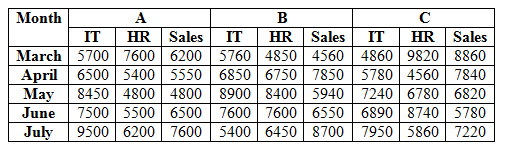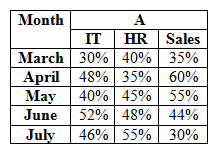# SBI Clerk Mains Quantitative Aptitude (Day-62)

Dear Aspirants, Our IBPS Guide team is providing new series of Quantitative Aptitude Questions for SBI Clerk Mains 2020 so the aspirants can practice it on a daily basis. These questions are framed by our skilled experts after understanding your needs thoroughly. Aspirants can practice these new series questions daily to familiarize with the exact exam pattern and make your preparation effective.

Start Quiz

Ensure Your Ability Before the ExamTake SBI Clerk 2020 Mains Free Mock Test

Missing Number Series

Direction (1-5): The questions below are based on the given Series-I. The series-I satisfy a certain pattern, follow the same pattern in Series-II and answer the questions given below.

1)

I) 32, 49, 75, 112, 162, 227

II) 1……196. If 196 is the nth term, what value should come in the place of (n – 1)th term.

A) 131

B) 142

C) 137

D) 148

E) 156

2)

I

3)

I) 24, 36, 72, 180, 540

II) 8…….630. If 630 is the nth term, what value should come in the place of (n – 1)th term.

A) 210

B) 180

C) 150

D) 120

E) 240

4)

I) 15, 136, 305,530, 819, 1180

II) 23…….1188. If 1188 is the nth term, what value should come in the place of (n – 2)th term.

A) 420

B) 538

C) 313

D) 827

E) 361

5)

I) 19, 47, 103, 215, 439, 887

II) 31—-631.If 631 is the nth term, what value should come in the place of (n + 1)th term.

A) 1268

B) 1234

C) 1271

D) 1256

E) 1220

Data Interpretation

Direction (6-10): Study the following information carefully and answer the questions given below.

The given table shows the number of employee works in three different companies in five different months.The given table shows the percentage of male employees in A in three different departments.6) The number of female HR employees in C in March is 25% more than the number of female HR employees in A in March. Number of male HR employees in C in March is what percent of the number of male HR employees in A in March?

A) 130.45%

B) 135.52%

C) 145.71%

D) 140.25%

E) 149.25%

7) If the number of female IT employees in C in June is 10% more than the number of female IT employees in A in June and the number of female IT employees in C in July is 40% of the total number of IT employees in C in July. Find the percentage decrease in the number of female IT employees in C from June to July?

A) 19.69%

B) 20.45%

C) 21.56%

D) 18.72%

E) 15.93%

8) What is the total number of female Sales employees in A in all the months together?

A) 16360

B) 17470

C) 16370

D) 17370

E) 15370

9) Out of 60% of total number of HR employees in B in March are regular employees and out of 40% of the total number of sales employees in A in April are regular employees. What is the difference between the regular HR employees in B in March and the regular sales employees in A in April?

A) 580

B) 630

C) 690

D) 530

E) 490

10) Out of 40% of the total number of sales employees in B in July are female and out of 25% of the total number of sales employees in C in April are female. What is the ratio of female sales employees in B in July to the number of female sales employees in C in April?

A) 87:49

B) 89:45

C) 96:47

D) 81:43

E) None of these

Directions (1-5) :

Series I,

32 + 42 + 1 = 49

49 + 52 + 1 = 75

75 + 62 + 1 = 112

112 + 72 + 1 = 162

162 + 82 + 1 = 227

Series II,

1 is 1st term

1 + 42 + 1 = 18

18 + 52 + 1 = 44

44 + 62 + 1 = 81

81 + 72 + 1 = 131(n – 1)th term

131 + 82 + 1 = 196

196 is nth term

Series I,

13 + (13 * 1) + 0 = 26

26 + (13 * 2) + 1 = 53

53 + (13 * 3) + 2 = 94

94 + (13 * 4) + 3 = 149

149 + (13 * 5) + 4 = 218

Series II,

29 is 1st term.

29 + (13 * 1) + 0 = 42

42 + (13 * 2) + 1 = 69

69 + (13 * 3) + 2 = 110

110 + (13 * 4) + 3 = 165 (nth term)

165 + (13 * 5) + 4 = 234 (n + 1)th term

Series I,

24 * 1.5 = 36

36 * 2 = 72

72 * 2.5 = 180

180 * 3 = 540

Series II,

8 is 1st term

8 * 1.5 = 12

12 * 2 = 24

24 * 2.5 = 60

60 * 3 = 180(n – 1)th term

180 * 3.5 = 630(nth term)

Series I,

15 + 112 = 136

136 + 132 = 305

305 + 152 = 530

530 + 172 = 819

819 + 192 = 1180

Series II,

23 + 112 = 144

144 + 132 = 313

313 + 152 = 538(n-2)th term

538 + 172 = 827

827 + 192 = 1188(nth term)

Series I,

19 * 2 + 9 = 47

47 * 2 + 9 = 103

103 * 2 + 9 = 215

215 * 2 + 9 = 439

439 * 2 + 9 = 887

Series II,

31 * 2 + 9 = 71

71 * 2 + 9 = 151

151 * 2 + 9 = 311

311 * 2 + 9 = 631(nth term)

631 * 2 + 9 = 1271(n + 1)th term

Directions (6-10) :

Number of female HR employees in A in March = 60/100 * 7600 = 4560

Number of male HR employees in A in March = 40/100 * 7600 = 3040

Number of female HR employees in C in March = 125/100 * 4560 = 5700

Number of male HR employees in C in March = 9820 – 5700 = 4120

Required percentage = 4120/3040 * 100 = 135.52%

Number of female IT employees in A in June = 7500 * 48/100 = 3600

Number of female IT employees in C in June = 3600 * 110/100 = 3960

Total number of IT employees in C in July = 7950

Number of female IT in C in July = 40/100 * 7950 = 3180

Required percentage = (3960 – 3180)/3960 * 100 = 19.69%

Number of female sales employees in A in march = 65/100 * 6200 = 4030

Number of female sales employees in A in April = 40/100 * 5550 = 2220

Number of female sales employees in A in May = 45/100 * 4800 = 2160

Number of female sales employees in A in June = 56/100 * 6500 = 3640

Number of female sales employees in A in July = 70/100 * 7600 = 5320

Required total = 4030 + 2220 + 2160 + 3640 + 5320 = 17370

Total number of HR employees in B in March = 4850

Regular HR employees in B in March = 60/100 * 4850 = 2910

Total number of sales employees in A in April = 5550

Regular sales employees in A in April = 40/100 * 5550 = 2220

Required difference = 2910 – 2220 = 690

Total number of sales employees in B in July = 8700

Number of female sales employees in B in July = 40/100 * 8700 = 3480

Total number of sales employees in C in April = 7840

Number of female sales employees in C in April = 25/100 * 7840 = 1960

Required ratio = 3480:1960

= 87:49

 Check Here to View SBI Clerk Mains 2020 Quantitative Aptitude Questions Day 61 Day 60 Day 59 Click Here for SBI Clerk 2020 – Detailed Exam Notification